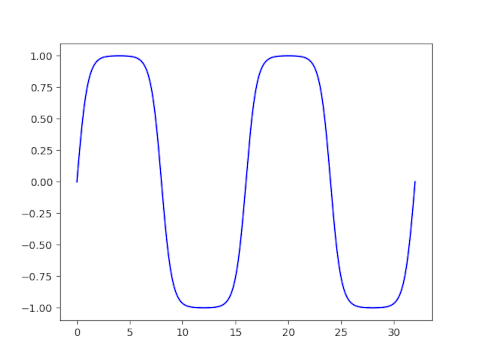# Solve for Jacobi sn parameter to have given period(s)

Here’s a calculation that I’ve had to do a few times. I’m writing it up here for my future reference and for the benefit of anybody else who needs to do the same calculation.

The Jacobi sn function is doubly periodic: it has one period as you move along the real axis and another period as you move along the imaginary axis. Its values are determined by a fundamental rectangle, and here we solve for a parameter 0 ≤ m < 1 that yields a desired length, or width, or aspect ratio for this rectangle.

You cannot specify both the length and width of the fundamental rectangle independently: you only have one degree of freedom. You can specify its length, or width, or aspect ratio.

This post focuses on sn, then explains how to modify the results for the other Jacobi elliptic functions—cn, dn, sc, etc.—at the end.

## Given period

A parameter value m corresponds to a horizontal period of 4K(m) and a vertical period of 2K(1-m) where K is the complete elliptic integral of the first kind.

K(m) is an increasing function from [0, 1) to [π/2, ∞). So the minimum horizontal period is 2π and the minimum vertical period is π.

## Given aspect ratio

The ratio of the horizontal period to the vertical period is given by

r(m) = 4K(m) / 2K(1-m) = 2K(m)/K(1-m).

The function r is an increasing function from [0, 1) to [0, ∞), so any aspect ratio is possible. This is important, for example, in conformal mapping. You cannot specify the length and width of the fundamental period independently, but you can solve for the parameter m that gives the aspect ratio you want, then follow it by a dilation to then get the length and width you want.

## Numerical computation

If you need to find m efficiently or to high precision, see . The code here simple and good enough for my purposes, and hopefully good enough for yours.

We need to either invert K or r, both of which are monotone functions, and so we can use the bisection method to find the value of m we’re after. The bisection method is not the most efficient, but it’s simple and very robust.

## Python code

```    from numpy import pi, linspace
from scipy.special import ellipk, ellipj
from scipy.optimize import bisect

def invert_ellipk(K):
"Given K, find m such that K(m) = K."
assert(K >= pi/2)
if K == pi/2:
return 0

# Find a bracket [a, b] for m
a = 0
b = 0.5
y = ellipk(b)
while y < K:
b = 0.5*(b + 1)
y = ellipk(b)
return bisect(lambda m: ellipk(m)-K, a, b)

def invert_horizontal_period(T):
"Find parameter m such that sn(z, m) has real period T."
return invert_ellipk(T/4)

def invert_vertical_period(T):
"Find parameter m such that sn(z, m) has imaginary period T."
return invert_ellipk(T/2)

def invert_r(r):
"Find parameter m such that the fundamental rectangle of sn(z, m) has aspect ratio r."

ratio = lambda m: 2*ellipk(m)/ellipk(1-m)

if r == 2:
return 1/2

a, b = 0.5, 0.5
while ratio(b) < r: b = 0.5*(1 + b) while ratio(a) > r:
a /= 2
return bisect(lambda m: r - ratio(m), a, b)
```

To test the code, let’s find m such that sn(z, m) has period 5 along the real axis.

```    import matplotlib.pyplot as plt

T = 16
m = invert_horizontal_period(T)
print(4*ellipk(m))
assert(abs(4*ellipk(m) - T) < 1e-8)
x = linspace(0, 2*T, 200)
plt.plot(x, sn(x, m))
```

This produces a plot that does have period 16.## Other Jacobi elliptic functions

There are 12 Jacobi elliptic functions, denoted with all pairs of {s, c, d, n} such that the two letters are different. The periods of the functions fall into three groups.

The functions sn, ns, cd, and dc all have real period 4K(m) and imaginary period 2K(1-m).

The functions cn, nc, ds, and sd all have real period 4K(m) and imaginary period 4K(1-m).

The functions cs, sc, dn, and nd all have real period 2K(m) and imaginary period 4K(1-m).

You can find plots of fundamental rectangles of these 12 functions here.

 Toshio Fukushima. Numerical computation of inverse complete elliptic integrals of the first and second kinds. Journal of Computational and Applied Mathematics. 249 (2013) 37–50.